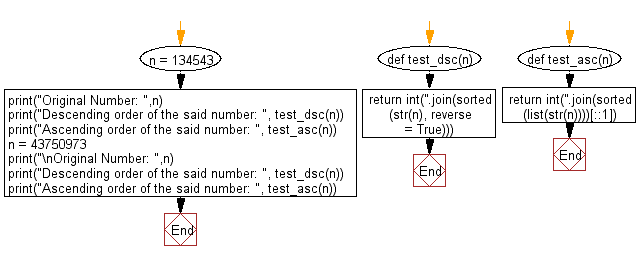﻿ Python: Sort a given positive number in descending/ascending order - w3resource# Python: Sort a given positive number in descending/ascending order

## Python List: Exercise - 214 with Solution

Write a Python program to sort a given positive number in descending/ascending order.

Descending -> Highest to lowest.
Ascending -> Lowest to highest

Sample Solution:

Python Code:

``````def test_dsc(n):
return int(''.join(sorted(str(n), reverse = True)))

def test_asc(n):
return int(''.join(sorted(list(str(n))))[::1])

n = 134543
print("Original Number: ",n);
print("Descending order of the said number: ", test_dsc(n));
print("Ascending order of the said number: ", test_asc(n));
n = 43750973
print("\nOriginal Number: ",n);
print("Descending order of the said number: ", test_dsc(n));
print("Ascending order of the said number: ", test_asc(n));
```
```

Sample Output:

```Original Number:  134543
Descending order of the said number:  544331
Ascending order of the said number:  133445

Original Number:  43750973
Descending order of the said number:  97754330
Ascending order of the said number:  3345779
```

Flowchart:## Visualize Python code execution:

The following tool visualize what the computer is doing step-by-step as it executes the said program:

Python Code Editor:

Have another way to solve this solution? Contribute your code (and comments) through Disqus.

Next: Write a Python program to merge two or more lists into a list of lists, combining elements from each of the input lists based on their positions.

What is the difficulty level of this exercise?

Test your Python skills with w3resource's quiz

﻿

## Python: Tips of the Day

Floor Division:

When we speak of division we normally mean (/) float division operator, this will give a precise result in float format with decimals.

For a rounded integer result there is (//) floor division operator in Python. Floor division will only give integer results that are round numbers.

```print(1000 // 300)
print(1000 / 300)```

Output:

```3
3.3333333333333335```